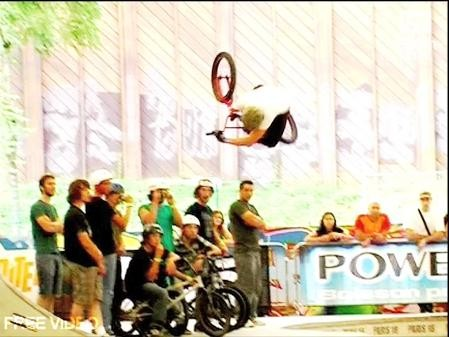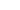invert alley-oop arial

• + 5stp-2 (Jul 29, 2008 at 15:14)
f*ckin ruiner^
• + 5chris1993 (Jul 29, 2008 at 15:23)
omg wat a dik ^^
• + 3shankmelank1 (Jul 27, 2008 at 11:33)
nice
• + 3chris1993 (Jul 29, 2008 at 15:10)
nice
• + 1giantrider03 (Nov 1, 2009 at 23:25)
nice
• + 3BrownBMX (Jul 27, 2008 at 14:57)
nice
• + 3minimanofmayhem (Aug 12, 2008 at 13:27)
nice
• + 3doms (Sep 6, 2008 at 6:45)
nice
• + 3downhiller4eva (Aug 19, 2008 at 9:09)
nice
• + 3doublerr (Jul 29, 2008 at 15:09)
nice
• + 3kennyomg (Jul 27, 2008 at 6:56)
nice
• - 1KonaKane (Nov 1, 2009 at 23:26)
thats nearly upside down!
• + 3benwalker2007 (Jul 28, 2008 at 0:41)
nice
• + 3Destry (Jul 27, 2008 at 23:05)
nice
• + 3alexbman (Jul 29, 2008 at 10:05)
nice
• + 3robbo2k7 (Jul 28, 2008 at 7:24)
nice
• + 3Torin-MH (Jul 27, 2008 at 15:47)
nice
• + 3luke1234567890 (Jul 28, 2008 at 14:49)
nice
• + 3canadaripper (Jul 28, 2008 at 7:25)
nice
• + 1nouser (Dec 1, 2008 at 7:22)
starting it back up, nice
• + 2silviubmx (Sep 10, 2008 at 13:39)
nice
• + 2RockGiant (Sep 7, 2008 at 4:54)
nice
• + 2hobbnobs (Sep 7, 2008 at 4:31)
nice
• + 2djforthewin (Sep 6, 2008 at 12:04)
nice
• + 1xankas (Feb 1, 2009 at 7:44)
nice
• + 1supersheekey (Nov 1, 2009 at 23:35)
snap!
• + 1dancharge (Aug 20, 2009 at 16:21)
nice
• + 1jeffrupert (Oct 3, 2009 at 15:35)
nice
• + 0frozenride (Aug 17, 2009 at 13:22)
f*ckin massive!! props• + 0fejoa123 (Sep 10, 2008 at 13:40)
lol ruined